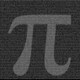# DFT - Dominant Cycle Period 8-50 bars - John Ehler

1447 visualizzazioni
This is the translation of discret cosine tranform ( DCT ) usage by John Ehler for finding dominant cycle period (DC).
The price is first filtered to remove aliasing noise(bellow 8 bars) and trend informations(above 50 bars), then the power is computed.
The trick here is to use a normalisation against the maximum power in order to get a good frequency resolution.
Current limitation in tradingview does not allow to display all of the periods, still the DC period is plot after beeing computed based on the center of gravity algo.

The DC period can be used to tune all of the indicators based on the cycles of the markets. For instance one can use this (DC period)/2 as an input for RSI .

Hope you find this of some interrest.
Note di rilascio: Fixing the High pass filter period. Now selectable.

## CommentiGreat script!
Below you can find a solution of plots limitation.
Hope this helps.

min = 8
l1 = hline(min + 0, color=color.new(#000000, 100), linewidth=0, linestyle=hline.style_dotted)
l2 = hline(min + 1, color=color.new(#000000, 100), linewidth=0, linestyle=hline.style_dotted)
l3 = hline(min + 2, color=color.new(#000000, 100), linewidth=0, linestyle=hline.style_dotted)
l4 = hline(min + 3, color=color.new(#000000, 100), linewidth=0, linestyle=hline.style_dotted)
l5 = hline(min + 4, color=color.new(#000000, 100), linewidth=0, linestyle=hline.style_dotted)
l6 = hline(min + 5, color=color.new(#000000, 100), linewidth=0, linestyle=hline.style_dotted)
l7 = hline(min + 6, color=color.new(#000000, 100), linewidth=0, linestyle=hline.style_dotted)
etc. up to l44 = hline(min + 43, color=color.new(#000000, 100), linewidth=0, linestyle=hline.style_dotted)
after that use fill function
Transperancy = 30
fill(l1, l2, color=setColor(DB_8, minDb,maxDb), transp=Transperancy)
fill(l2, l3, color=setColor(DB_9, minDb,maxDb), transp=Transperancy)
fill(l3, l4, color=setColor(DB_10, minDb,maxDb), transp=Transperancy)
fill(l4, l5, color=setColor(DB_11, minDb,maxDb), transp=Transperancy)
fill(l5, l6, color=setColor(DB_12, minDb,maxDb), transp=Transperancy)
fill(l6, l7, color=setColor(DB_13, minDb,maxDb), transp=Transperancy)
fill(l7, l8, color=setColor(DB_14, minDb,maxDb), transp=Transperancy)
fill(l8, l9, color=setColor(DB_15, minDb,maxDb), transp=Transperancy)
fill(l9, l10, color=setColor(DB_16, minDb,maxDb), transp=Transperancy)
etc. up to fill(l43, l44, color=setColor(DB_50, minDb,maxDb), transp=Transperancy)
Rispondi